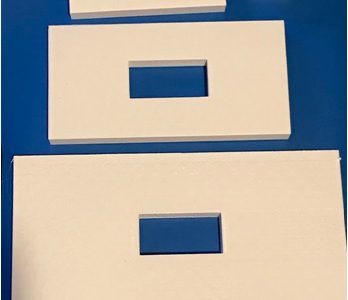# Area, Perimeter and Ratio ToolWe are proposing a tool that will help students determine area and perimeter. Our tool will consist of rectangular 2D frames.  The dimensions of the whole figure (side lengths) will be a ratio of the inner frames dimensions (1:2, 1:3, etc.).  Our goal is to have students realize the relationships between perimeter and area when the dimensions are changed.  What happens to the area and perimeter of a figure when a figure’s sides are cut in half (or doubled)? In thirds (or tripled)? Students will also be able to practice calculating area and perimeter.

Students will be able to physically hold the figure and count the lengths of the sides, allowing the mind and body to be fully engaged while calculating perimeter and area.  Students will be able to take multiple frames in order to draw more meaningful connections about perimeter and area, and the relationships created between figures and their ratios.

We believe our tool will allow students to have a great hands-on experience.  They will not only be able to visualize, but will be able to physically manipulate the tool while forming thoughts and connections about area and perimeter.  The physical nature of the tool and the manipulation that will occur will help a child’s fine motor skills.  Instead of just listening to steps and memorizing sequences, the children will ideally be able to understand in a deeper context the relationship between altering a figure’s dimensions and what that means for the perimeter and area.

If our tool is successful, the students using it would attain knowledge about side lengths, perimeter and area.  If our project was successful, it would mean the relationships between side length, area and perimeter would be clearly found, recognized and understood by students.  For example, students would see that cutting the sides of the original frame into thirds would create an equal ratio for the perimeter (1:3), but the area would be a 1:9 ratio.

If our tool were to fail to work, this would mean that we may have a misconception about the relationship between dimensions of a figure and area and perimeter.  If our tool failed, it would mean that it was not designed properly to show an accurate relationship between area, perimeter and side lengths.

To test out our design, we could give students pictures of shapes and ask them to find the perimeter and area.  These formulas could be provided, depending on level of students.  We could then ask the students to determine relationships between the original figure, and figures that have a half or a third of its side lengths.  If they can figure out the relationships and ratios, we could then discuss why these relationships occur.